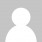# BEC-Difficulty Levels

• This topic has 1 reply, 2 voices, and was last updated 9 years, 11 months ago byAnonymous.
• Creator
Topic

I just took my last test today and like everybody I started analyzing how I did right after. I was surprised and slightly disappointed at the amount of theory questions I got versus mathematical. Believe or not I actually succeed at the mathematical questions. One the first testlet (medium) I received about 75% theory. That was the same for the second and third. My conclusion is that the second and third teslet were medium difficulty as well.

So in short my questions is this. Can you tell the difficulty of the testlet by the amount of mathematical question you receive versus theory questions?

FAR 10/10/12~~~~~~87
REG 11/20/12~~~~~~80
AUD 01/08/13~~~~~~79
BEC 02/22/13~~~~~~81

Viewing 1 replies (of 1 total)
• Author
RepliesAnonymous
Inactive

No. Computation and theory questions have nothing to do with the weight of the question. It depends on the topic and the depth of knowledge needed to answer it correctly.

Viewing 1 replies (of 1 total)
`<a class="" href="" title="" rel="" style="" download="" target=""> <abbr> <blockquote class="" style="" cite=""> <div class="" style=""> <span class="" style=""> <code class="" style=""> <pre class="" style=""> <em class="" style=""> <i class="" style=""> <b class="" style=""> <strong class="" style=""> <del datetime="" class="" style=""> <h1 align="" class="" style=""> <h2 align="" class="" style=""> <h3 align="" class="" style=""> <h4 align="" class="" style=""> <h5 align="" class="" style=""> <h6 align="" class="" style=""> <ul class="" style=""> <ol class="" style="" start=""> <li class="" style=""> <img class="" style="" src="" border="" alt="" height="" width=""> <table align="" bgcolor="" border="" class="" style=""> <tbody align="" valign="" class="" style=""> <td align="" valign="" class="" style=""> <tfoot align="" valign="" class="" style=""> <th align="" valign="" class="" style=""> <thead align="" valign="" class="" style=""> <tr align="" valign="" class="" style=""> `